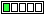All about flooble | fun stuff | Get a free chatterbox | Free JavaScript | Avatarsperplexus dot infoInequality (Posted on 2006-08-03)let Z(n)=Ln(n)-(1/2 + ... + 1/n)

Prove that 0 < Z(n) < 1-1/n, where n is an integer greater then one (Ln(n) being naturalbase logarithm of n)

 No Solution Yet Submitted by atheron No RatingComments: ( Back to comment list | You must be logged in to post comments.)Visual Integral Calculus| Comment 2 of 3 |This can be vizualized almost immediately once the inequality is written in the equivalent form

1/2+1/3+...+1/n < Ln(n) < 1+1/2+...+1/(n-1)

since Ln(n) is the integral from 1 to n of dx/x and the sums on the left and right respectively underestimate and overestimate the integral.

A fancy proof can be given based on integrating from k to k+1 the inequality

1/(k+1) < 1/x  < 1/k

that holds for x in the open interval (k,k+1) to yield

1/(k+1) < integral from k to k+1 of dx/x < 1/k. Then summing from k=1 to n-1 gives the desired result.

Edited on August 5, 2006, 3:11 am
 Posted by Richard on 2006-08-03 21:04:08Please log in:

 Search: Search body:
Forums (0)